# Chapter 5: Continuous Random Variables¶

This Jupyter notebook is the Python equivalent of the R code in section 5.9 R, pp. 253 - 255, Introduction to Probability, Second Edition, Blitzstein & Hwang.

In :
import numpy as np


## Python, SciPy and Matplotlib¶

In this section we will introduce continuous distributions in Python and SciPy, learn how to make basic plots, demonstrate the universality of the Uniform by simulation, and simulate arrival times in a Poisson process.

## Uniform, Normal, and Exponential distributions¶

For continuous distributions in scipy.stats, the pdf function gives the PDF, the cdf function gives the CDF, and the rvs function generates random numbers from the continuous distribution. This is in keeping with the application programming interface of the discrete statistical distributions in scipy.stats. Thus, we have the following functions:

### Uniform¶

scipy.stats.uniform provides functions for Uniform continuous random variables.

• To evaluate the $Unif(a, b)$ PDF at $x$, we use uniform.pdf(x, a, b).
• For the CDF, we use uniform.cdf(x, a, b).
• To generate $n$ realizations from the $Unif(a, b)$ distribution, we use uniform.rvs(a, b, size=n).
In :
# seed the random number generator
np.random.seed(1597)

from scipy.stats import uniform

#print(uniform.__doc__)

a = 0
b = 4
x = 3
n = 10

print('PDF of Unif({}, {}) evaluated at {} is {}\n'.format(a, b, x, uniform.pdf(x, a, b)))

print('CDF of Unif({}, {}) evaluated at {} is {}\n'.format(a, b, x, uniform.cdf(x, a, b)))

print('Generating {} realizations from Unif({}, {}):\n{}'.format(n, a, b, uniform.rvs(a, b, size=n)))

PDF of Unif(0, 4) evaluated at 3 is 0.25

CDF of Unif(0, 4) evaluated at 3 is 0.75

Generating 10 realizations from Unif(0, 4):
[3.24085002 2.48793265 0.69337744 3.98212677 3.53906749 0.19890551
3.30252222 2.37991343 1.42342695 1.92222338]


### Normal¶

scipy.stats.norm provides functions for normal continuous random variables.

• To evaluate the $N(\mu, \sigma^2)$ PDF at $x$, we use norm.pdf(x, loc, scale), where the parameter loc corresponds to $\mu$ and scale corresponds to standard deviation $\sigma$ (and not variance $\sigma^2$).
• For the CDF, we use norm.cdf(x, loc, scale).
• To generate $n$ realizations from the $N(\mu, \sigma^2)$ distribution, we use norm.rvs(loc, scale, size=n).
In :
np.random.seed(2584)

from scipy.stats import norm

#print(norm.__doc__)

mu = 0.0
sigma = 2.0
x = 1.5

print('PDF of N({}, {}) evaluated at {} is {}\n'.format(mu, sigma, x, norm.pdf(x, mu, sigma)))

print('CDF of N({}, {}) evaluated at {} is {}\n'.format(mu, sigma, x, norm.cdf(x, mu, sigma)))

print('Generating {} realizations from N({}, {}):\n{}'.format(n, mu, sigma, norm.rvs(mu, sigma, size=n)))

PDF of N(0.0, 2.0) evaluated at 1.5 is 0.15056871607740221

CDF of N(0.0, 2.0) evaluated at 1.5 is 0.7733726476231317

Generating 10 realizations from N(0.0, 2.0):
[-4.56890553  1.4163793  -0.72812069  1.80610792 -1.5542524   0.4551897
-1.82794816  1.58959742 -3.26902153 -2.71002864]


☣ 5.9.1 (Normal parameters in scipy.stats.norm). Note that we have to input the standard deviation for the scale parameter value, not the variance! For example, to get the $N(10, 3)$ CDF at 12, we usenorm.cdf(12, 10, np.sqrt(3)). Ignoring this is a common, disastrous coding error.

In :
loc = 10
var = 3
scale = np.sqrt(var)
x = 12

ans = norm.cdf(x, loc, scale)

print('N({}, {}) CDF at {} is {}'.format(loc, scale, x, ans))

N(10, 1.7320508075688772) CDF at 12 is 0.8758934605050381


### Exponential¶

scipy.stats.expon provides functions for exponential continuous random variables. The probability density function for $Expo(\lambda)$ is:

\begin{align} f(x) &= e^{-x} \end{align}

The probability density is defined in the “standardized” form. To shift and/or scale the distribution use the loc and scale parameters. Specifically, expon.pdf(x, loc, scale) is identically equivalent to expon.pdf(y) / scale with y = (x - loc) / scale.

• To evaluate the $Expo(\lambda)$ PDF at $x$, we use expon.pdf(x, scale=1/lambda), where the $\lambda$ corresponds to scale=1/lambd.
• For the CDF, we use expon.cdf(x, scale=1/lambd).
• To generate $n$ realizations from the $Expo(\lambda)$ distribution, we use expon.rvs(scale=1/lambd, size=n).
In :
np.random.seed(4181)

from scipy.stats import expon

#print(expon.__doc__)

lambd = 2.1
x = 5

expon.pdf(x, scale=1/lambd)

print('PDF of Expo({}) evaluated at {} is {}\n'.format(lambd, x, expon.pdf(x, scale=1/lambd)))

print('CDF of Expo({}) evaluated at {} is {}\n'.format(lambd, x, expon.cdf(x, scale=1/lambd)))

print('Generating {} realizations from Expo({}):\n{}\n'.format(n, lambd, expon.rvs(scale=1/lambd, size=n)))

PDF of Expo(2.1) evaluated at 5 is 5.782654363446904e-05

CDF of Expo(2.1) evaluated at 5 is 0.9999724635506503

Generating 10 realizations from Expo(2.1):
[0.44907946 0.77554149 1.469014   0.15728342 0.92258566 0.54294386
0.80347768 0.23157762 0.34759354 1.03891708]



Due to the importance of location-scale transformations for continuous distributions, SciPy has default parameter settings for each of these three families. The default for the Uniform scipy.stats.uniform is loc=0, scale=1, the default for the Normal scipy.stats.norm is loc=0, scale=1, and the default for the Exponential scipy.stats.expon is loc=0, scale=1.

For example, uniform.pdf(0.5), with no additional inputs, evaluates the $Unif(0, 1)$ PDF at 0.5.

In :
uniform.pdf(0.5)

Out:
1.0

norm.rvs(size=10), with no additional inputs, generates 10 realizations from the $N (0, 1)$ distribution.

In :
norm.rvs(size=10)

Out:
array([ 0.79839927, -0.69769073,  0.34055804, -0.22043838,  0.79782274,
-2.60292799, -1.53866926,  0.93084378,  0.81590117, -0.42043989])

This means there are two ways to generate a $N(\mu, \sigma^2)$ random variable in SciPy. After choosing our values of $\mu$ and $\sigma$,

In :
mu = 1
sigma = 2


we can do either of the following:

In :
ans1 = norm.rvs(mu, sigma, size=1, random_state=42)
ans2 = mu + sigma * norm.rvs(size=1, random_state=42)

print('norm.rvs(mu, sigma, size=1) = {}'.format(ans1))
print('mu + sigma * norm.rvs(size=1) = {}'.format(ans2))

norm.rvs(mu, sigma, size=1) = [1.99342831]
mu + sigma * norm.rvs(size=1) = [1.99342831]


Either way, we end up generating a draw from the $N(\mu, \sigma^2)$ distribution.

## Plots in Matplotlib¶

Plotting in Python within a Jupyter notebook is done in Matplotlib.

First, we import matplotlib.pyplot module. To cut down on typing, we can alias that imported matplotlib.pyplot module reference as plt for short. We then invoke the %matplotlib inline% Jupyter notebook magic command in order to create Matplotlib graphs right in your notebook.

In :
import matplotlib.pyplot as plt

%matplotlib inline


Here is how we can create a plot of the standard Normal PDF from −3 to 3 using Matplotlib in Jupyter notebook.

numpy.linspace creates an evenly-spaced numerical sequence, ranging from start to stop. The third function parameter num lets you specify how many numbers in the sequence to generate.

We use numpy.linspace(-3, 3, 1000) to generate 1000 numbers ranging from -3 to 3 as our x values in the graph; with 1000 values in the sequence, the curve looks very smooth. If we were to choose num=20, the piecewise linearity would become very apparent.

In :
x = np.linspace(-3, 3, 1000)


We can then call norm.pdf(x) to generate the y values in the graph, and pass both the x and y values to matplotlib.pyplot.plot. This will render a graph of $N(0,1)$ from -3 to 3. pyplot.show will display the graph.

In :
plt.plot(x, norm.pdf(x))
plt.show()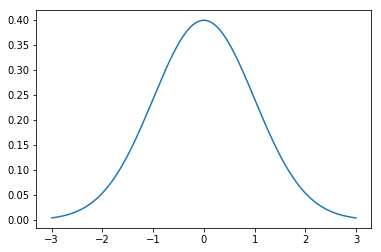Alternately, we could "freeze" an instance of the scipy.stats.norm distribution object to fix the shape, location and scale parameters. This might be useful if you are working with multiple distributions with differing shape, location and scale, and wanted to use these distributions throughout your notebook.

In :
rv1 = norm(0, 1)
rv2 = norm(0.5, 1)
rv3 = norm(1, 1)

plt.plot(x, rv1.pdf(x), label='norm(0, 1)')
plt.plot(x, rv2.pdf(x), label='norm(0.5, 1)')
plt.plot(x, rv3.pdf(x), label='norm(1, 1)')

plt.legend()

plt.show()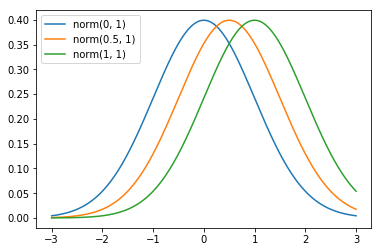We can also set the axis labels and plot title with pyplot.xlabel, pyplot.ylabel, and pyplot.title functions.

In :
rv = norm()
plt.plot(x, rv.pdf(x))

plt.xlabel('x')
plt.ylabel('norm.pdf(x)')
plt.title('Standard Normal PDF')

plt.show()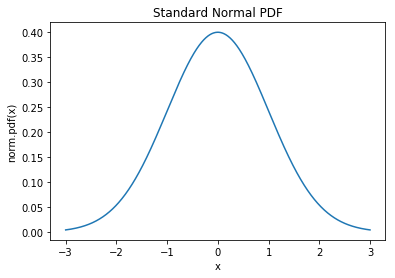The axis limits can be set manually with pyplot.xlim and pyplot.ylim If, for example, you wanted the vertical axis to range from 0 to 1, you would follow the call to pyplot.plot with pyplot.ylim([0, 1]).

In :
plt.plot(x, rv.pdf(x))
plt.ylim([0, 1])
plt.show()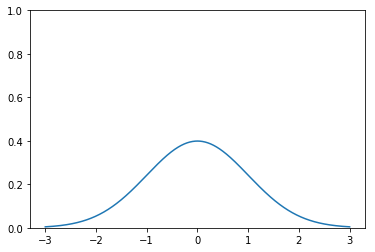Finally, to change the color of the plot, add color="orange" or color="green", or whatever your favorite color is! (see Specifying Colors in the Matplotlib documentation)

In :
plt.plot(x, rv.pdf(x), color='orange')
plt.show()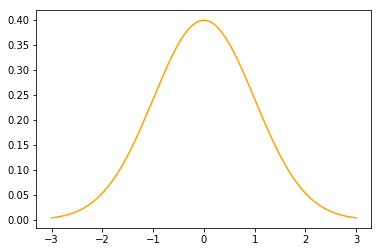## Universality with Logistic¶

We proved in Example 5.3.4 that for $U \sim Unif(0, 1)$, the r.v. $log\left(\frac{U}{1−U}\right)$ follows a Logistic distribution. In SciPy and uniform.rvs, we can simply generate a large number of $Unif(0, 1)$ realizations and transform them.

In :
u = uniform.rvs(size=10**4)
x = np.log(u/(1-u))
x

Out:
array([ 2.0197625 , -0.72108035, -1.86938026, ..., -1.2638552 ,
1.8532956 ,  1.46624836])

Now x contains 104 realizations from the distribution of $log\left(\frac{U}{1−U}\right)$. We can visualize them with a histogram, using matplotlib.hist. The histogram resembles a Logistic PDF, which is reassuring. To control how fine-grained the histogram is, we can set the number of bins in the histogram via the bins parameter (the 2nd parameter passed into pyplot.hist: hist(x, 100) produces a finer histogram, while hist(x, 10) produces a coarser histogram.

To illustrate, we will generate two graphs side-by-side with pyplot.figure, and use a pyplot.subplot for each graph.

In :
fig = plt.figure(figsize=(14, 6))

ax1.hist(x, 100)
ax1.set_title('bins=100')

ax2.hist(x, 10)
ax2.set_title('bins=10')

plt.show()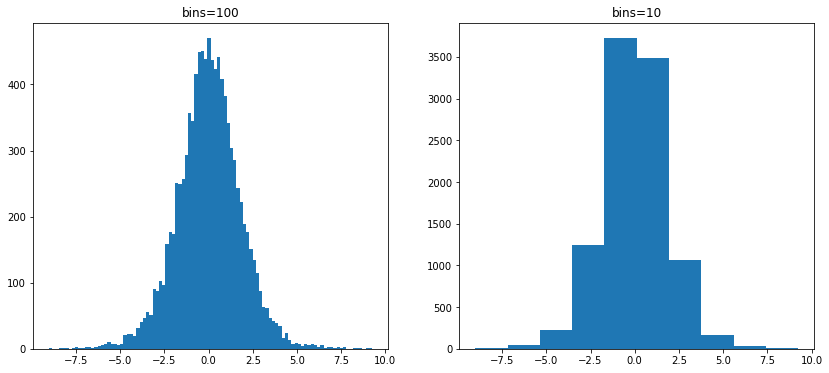## Poisson process simulation¶

To simulate $n$ arrivals in a Poisson process with rate $\lambda$, we first generate the interarrival times as i.i.d. Exponentials and store them in variable x:

In :
n = 50
lambd = 10
x = expon.rvs(scale=1/lambd, size=n)
print(x)

[0.17627964 0.01902565 0.02146015 0.19802154 0.16173354 0.02476036
0.02394206 0.11207592 0.00051518 0.09787187 0.06122226 0.07313865
0.07720919 0.15936267 0.00195037 0.01965782 0.00122744 0.03186262
0.13671518 0.29913587 0.05325137 0.1329256  0.18825129 0.31339715
0.07390052 0.2792474  0.19905106 0.1237123  0.12739159 0.05063309
0.00787248 0.20327465 0.28319828 0.0039541  0.0084741  0.01842843
0.00354277 0.08189181 0.07338873 0.03824727 0.02011739 0.0211869
0.02824106 0.07150573 0.24463567 0.35755971 0.01220348 0.00555063
0.05732313 0.20553653]


Then we convert the interarrival times into arrival times using the numpy.cumsum function, which stands for "cumulative sum".

In :
t = np.cumsum(x)
print(t)

[0.17627964 0.19530529 0.21676545 0.41478698 0.57652053 0.60128088
0.62522295 0.73729887 0.73781405 0.83568592 0.89690818 0.97004683
1.04725602 1.20661869 1.20856907 1.22822689 1.22945433 1.26131695
1.39803213 1.697168   1.75041936 1.88334496 2.07159625 2.38499339
2.45889391 2.73814132 2.93719238 3.06090468 3.18829627 3.23892936
3.24680184 3.4500765  3.73327478 3.73722888 3.74570298 3.76413141
3.76767418 3.849566   3.92295473 3.961202   3.98131938 4.00250628
4.03074735 4.10225307 4.34688874 4.70444845 4.71665193 4.72220256
4.77952569 4.98506222]


The array t now contains all the simulated arrival times.

Joseph K. Blitzstein and Jessica Hwang, Harvard University and Stanford University, © 2019 by Taylor and Francis Group, LLC